# Math in Focus Grade 6 Chapter 1 Lesson 1.4 Answer Key Squares and Square Roots

Go through the Math in Focus Grade 6 Workbook Answer Key Chapter 1 Lesson 1.4 Squares and Square Roots to finish your assignments.

## Math in Focus Grade 6 Course 1 A Chapter 1 Lesson 1.4 Answer Key Squares and Square Roots

### Math in Focus Grade 6 Chapter 1 Lesson 1.4 Guided Practice Answer Key

Find the square of each number.

Question 1.
2
2 x 2 = 4

Explanation:
The square of 2 is 4.

Question 2.
6
6 x 6 = 36

Explanation:
The square of 6 is 36.

Question 3.
9
9 x 9 = 81

Explanation:
The square of 9 is 81.

Question 4.
11
11 x 11 = 121

Explanation:
The square of 11 is 121.

Find a square root of a perfect square.

a) A square has an area of 9 square inches. Find the length of each side of the square.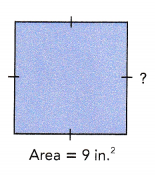You know that
Area of square = length × length.
To find the length of a side of the square, you need to find the number whose square is 9.
Recalling the multiplication facts of 3, you know that 3 × 3 = 9.
So, the length of each side of the square is 3 inches.
3 is called a square root of 9. This can be written as $$\sqrt{9}$$ = 3.
You read this as “the square root of 9 equals 3”.b) Find the square root of 100.

I can relate this to finding the length of the side of a square, given that it has an area of 1oo units2.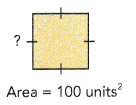Method 1
Recalling the multiplication facts of 10, you know that
10 × 10 = 100
So, $$\sqrt{100}$$ = 10.

Method 2
By prime factorization,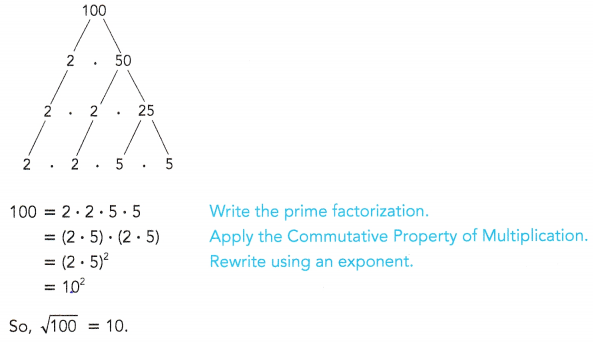Find the square root of each number.

Question 5.
25
5

Explanation:
Recalling the multiplication facts of 25, you know that
25 = 5 x 5
= 5²
So, $$\sqrt{25}$$ = 5

Question 6.
64
8

Explanation:
Recalling the multiplication facts of 64, you know that
64 = 8 x 8
= 8²
So, $$\sqrt{64}$$ = 8

Question 7.
144
12

Explanation:
Recalling the multiplication facts of 144, you know that
144 = 12 x 12
= 12²
So, $$\sqrt{144}$$ = 12

Question 8.
196
13

Explanation:
Recalling the multiplication facts of 169, you know that
169 = 13 x 13
= 13²
So, $$\sqrt{169}$$ = 13

### Math in Focus Course 1A Practice 1.4 Answer Key

Find the square of each number.

Question 1.
3
3 x 3 = 9

Explanation:
The square of 3 is 9.

Question 2.
7
7 x 7 = 49

Explanation:
The square of 7 is 49.

Question 3.
12
144

Explanation:
The square of 12 is 144.

Question 4.
10
100

Explanation:
The square of 10 is 100.

Find the square root of each number.

Question 5.
36
6

Explanation:
Recalling the multiplication facts of 36, you know that
36 = 6 x 6
= 6²
So, $$\sqrt{36}$$ = 6

Question 6.
81
9

Explanation:
Recalling the multiplication facts of 81, you know that
81 = 9 x 9
= 9²
So, $$\sqrt{81}$$ = 9

Question 7.
121
11

Explanation:
Recalling the multiplication facts of 121, you know that
121 = 11 x 11
= 11²
So, $$\sqrt{121}$$ = 11

Question 8.
49
7

Explanation:
Recalling the multiplication facts of 49, you know that
49 = 7 x 7
= 7²
So, $$\sqrt{49}$$ = 7

Solve.

Question 9.
List the perfect squares that are between 25 and 100.

Find the value of each of the following.

Question 10.
352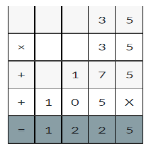35 x 35 = 1225

Explanation:
The square of 35 is 1225.

Question 11.
562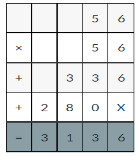56 x 56 = 3136

Explanation:
The square of 56 is 3136.

Question 12.
642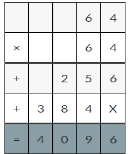64 x 64 = 4096

Explanation:
The square of 64 is 4096.

Question 13.
$$\sqrt{289}$$
17

Explanation:
Recalling the multiplication facts of 289, you know that
289 = 17 x 17
= 17²
So, $$\sqrt{289}$$ = 17

Question 14.
$$\sqrt{400}$$
20

Explanation:
Recalling the multiplication facts of 400, you know that
400 = 20 x 20
= 20²
So, $$\sqrt{400}$$ = 20

Question 15.
$$\sqrt{484}$$
22

Explanation:
Recalling the multiplication facts of 484, you know that
484 = 22 x 22
= 22²
So, $$\sqrt{484}$$ = 22

Solve.

Question 16.
Given that 412 = 1,681, find the square of 410.
168100

Explanation:
If 412 = 1,681 then 4102 = 168100.

Question 17.
Given that 512 = 2,601, find the square root of 260,100.
510

Explanation:
If 512 = 2601 then the square root of 260100 will be 510.

Question 18.
Given that $$\sqrt{676}$$ = 26, evaluate $$\sqrt{2,704}$$.
52

Explanation:
Recalling the multiplication facts of 400, you know that
2704 = 52 x 52
= 52²
So, $$\sqrt{2704}$$ = 52

Question 19.
Given that $$\sqrt{1,521}$$ = 39, evaluate 3902.
152100

Explanation:
If $$\sqrt{1,521}$$ = 39 then 390² = 152100.

Question 20.
Heather wants to make a giant square quilt with sides of length 28 feet. She uses square patches of fabric that have sides of length 4 feet. How many patches of fabric will Heather need to make the giant square quilt?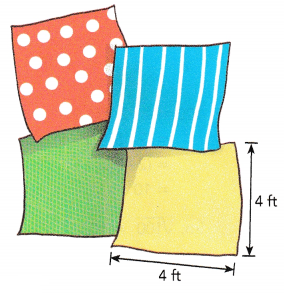7 patches of fabric Heather will need to make the giant square quilt.

Explanation:
Heather wants to make a giant square quilt with sides of length 28 feet.
She uses square patches of fabric that have sides of length 4 feet.
4 x 7 = 28
So, 7 patches of fabric Heather will need to make the giant square quilt.

Question 21.
This week, customers at a carpet store pay $3 for a square foot of carpet. Next week the store will be having a sale. During the sale, each square foot of carpet will cost only$2. Neil wants to carpet two square rooms in his house. The floor in one room is 10 feet by 10 feet. The floor in the other room is 14 feet by 14 feet. How much money will Neil save if he waits to buy carpet during the sale?